International
Tables for
Crystallography
Volume H
Powder diffraction
Edited by C. J. Gilmore, J. A. Kaduk and H. Schenk

International Tables for Crystallography (2018). Vol. H, ch. 1.1, pp. 15-19

Section 1.1.5. The background

R. E. Dinnebiera* and S. J. L. Billingeb,c

aMax-Planck-Institute for Solid State Research, Heisenbergstrasse 1, D-70569 Stuttgart, Germany,bDepartment of Applied Physics and Applied Mathematics, Columbia University, 500 West 120th Street, Room 200 Mudd, MC 4701, New York, NY 10027, USA, and cCondensed Matter Physics and Materials Science Department, Brookhaven National Laboratory, PO Box 5000, Upton, NY 11973–5000, USA
Correspondence e-mail:  r.dinnebier@fkf.mpg.de

1.1.5. The background

| top | pdf |

1.1.5.1. Information content in the background

| top | pdf |

As discussed above, the elastic scattering from a crystalline powder consists of sharp rings, or peaks, of scattering at the 2θ angles where the Bragg or von Laue laws are satisfied. In general these sharp peaks sit on top of a background' which is broad and somewhat featureless. There are two components to this background, illustrated in Fig. 1.1.1: extraneous counts in the detector from things other than the sample, and non-Bragg scattering from the sample itself. The former are rarely of interest scientifically and the objective of a good experimental design is to minimize them as far as possible, or explicitly measure and subtract them, and then account well in any model or data interpretation for the part that cannot be eliminated from the measurement. Historically, the diffuse-scattering signals from the sample itself were also considered to be an inconvenience to be minimized and removed, and indeed in many cases this is still the best course of action (for example, sample fluorescence can be eliminated by choosing to work at an X-ray energy that lies below the absorption edge of a constituent atom). However, the diffuse background' from the sample can contain crucial information about defects, disorder and nanoscale order in the sample, and increasingly we are interested in studying it in order to understand the properties of the material that is under investigation. In some cases, such as glasses, liquids and samples of small nanoparticles, there is no Bragg scattering at all and only a diffuse scattering signal (see Chapter 5.6).

All the intensity scattered by the sample can be categorized as either coherent or incoherent and as elastic or inelastic, which are defined as follows. The coherency of the signal derives from whether or not the scattered waves interfere with each other constructively, and the resulting intensities are different in each case. For coherent scattering, the waves contributing to the signal are all summed first, before the wave amplitude is squared, to find the intensity distribution, which is the modulus squared of the resulting wave. For incoherent waves, one simply squares the amplitude of each wave to get its intensity and sums these together to get the total intensity. Switching to a consideration of the elasticity of the scattering, we define the scattering as elastic if the incident and scattered waves have the same energy, in which case no energy was exchanged during the scattering process between the incident wave and the sample, and inelastic scattering as the opposite. Inelastic scattering may result in a gain or a loss of energy of the scattered particle depending on the nature of the scattering, which results in a change in the wavelength of the scattered particle. There are also some non-scattering processes that can take place, such as absorption and fluorescence, but emissions resulting from these processes can also be categorized by whether or not they are coherent and elastic. It should be noted that the total energy of the system must be conserved during the scattering process, and so when a scattered wave gains or loses energy it exchanges it with the sample. This is used as a way of probing excitations in a material. Table 1.1.1summarizes many of the types of diffuse scattering coming from a sample and categorizes them by their coherency and elasticity.

 Table 1.1.1| top | pdf | Types of scattering from a sample
Type of scatteringCoherentIncoherent
Elastic Bragg scattering Laue monotonic diffuse scattering
Magnetic Bragg scattering Neutron incoherent scattering
Bragg scattering from ferroelectric/magnetic order Multiple scattering (incoherent)
Diffuse scattering from static defects
Diffuse signal from small nanoparticles (<10 nm)
Scattering from amorphous material (except excitations)
Multiple scattering (coherent)
Inelastic Thermal diffuse scattering Compton scattering
Spin-wave scattering Fluorescence
Paraelectric/paramagnetic scattering Incoherent scattering from hydrogen
Scattering from liquids

1.1.5.2. Background from extraneous sources

| top | pdf |

The most commonly observed extraneous, or parasitic, scattering is from the sample container (such as a capillary) that holds the sample during the measurement. Another large contribution may come from air scattering, which originates principally from scattering of the direct beam by molecules in the air in the beam path, both before and after the sample. Air-scattering effects can be minimized by enclosing as much of the beam path as possible in a tube which may be evacuated or where the air is replaced by a weakly scattering gas (such as He in the case of X-rays). Air scattering that is detected by the detector can also be reduced by careful collimation of the beams and then shielding the detector from detecting radiation that does not originate from the sample position. Collimating the incident beam is straightforward and results in a big reduction in air scattering. For point detectors it is also straightforward to collimate the scattered beam, but the modern trend towards using linear and area detectors makes this more difficult. There is sometimes a trade-off between collimating the scattered beam to reduce background and having uniform backgrounds that do not vary with angle because of incomplete angle-dependent collimation. Incomplete angle-dependent collimation can be very difficult to correct when trying to measure diffuse scattering quantitatively and the current trend is to have minimal secondary collimation.

There is increasing interest in carrying out in situ experiments under extreme conditions of pressure, temperature, magnetic field and so on (see Chapters 2.6to 2.8). These experiments inevitably introduce additional scattering from the environment. Again, there is a balance between finding creative ways to reduce these backgrounds, and simply making them less problematic in the data analysis. For example, in a diamond-anvil cell, where the beam accesses the sample through the diamond, one can drill a hole part way through the diamond to accommodate the direct beam and make the direct beam small enough to fit in the hole. This increases the complexity of the measurement as alignment becomes harder, but it is usually worth it. Shielding structural parts of the environment cell with an absorbing material, such as lead for X-rays or a borated material for neutrons, can help to reduce unwanted background intensity a lot, as can making thin, transparent windows for the incident and scattered beams.

An additional source of background in the signal does not come from scattering at all, but from electrical noise in the detector electronics. For some types of detectors it may be important to measure dark' exposures with the X-rays turned off and subtract these carefully from the experimental data. It is also possible to detect signals from cosmic rays, which can leave tracks in two-dimensional detector signals.

| top | pdf |

1.1.5.3.1. Elastic coherent diffuse scattering

| top | pdf |

As discussed in Section 1.1.4.1.1, decreasing the size of a crystal leads to an increase in the width of the Bragg peaks. When the size of the crystallite becomes very small, as a rule of thumb below 10 nm in diameter for typical unit cells, the widths of the Bragg peaks become so large that they merge and overlap, and it does not make sense to use delta-function Bragg peaks as the starting point for the analysis. At this point the coherent diffraction is completely diffuse in nature. Nonetheless, it still contains structural information. To see this we begin again with the Laue equation before we assumed periodicity [equation (1.1.39)]. For the simple case of a diatomic gas such as N2, the sum would be taken only over two atoms, since scattering from a single molecule will be coherent but that from different molecules will be incoherent. In that case we have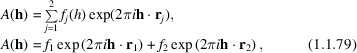and the intensity is proportional to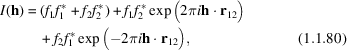where r12 = r1r2. For a diatomic molecule where both atoms are the same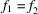and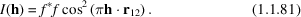The scattering from a diatomic molecule of an element is simply a single-component cosine wave with a wavelength that depends on the separation of the atoms in the molecule. In an actual experiment there will be scattering from all the molecules that have every orientation with equal probability, so it is necessary to take an orientational average of the scattering. How this is done is shown in Chapter 5.7on PDF analysis, but the result is the Debye equation (Debye, 1915),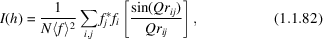where N is the total number of atoms. For our diatomic molecule this becomes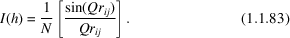For clusters of atoms such as larger molecules or small nanoparticles that are intermediate in size between a diatomic molecule and a small chunk of crystal, the Debye equation is exact and may be used to calculate the intensity of the scattering. As the clusters get larger and the structure more periodic, such as small chunks of crystal, the scattering calculated from the Debye equation crosses smoothly to that obtained from the periodic Laue equation. The finite size broadened crystallographic model works well as a starting point for calculating scattering from well ordered crystals down to nanoparticle sizes of 10 nm, but loses accuracy rapidly below this particle size. The Debye equation is accurate for all particle sizes, but becomes computationally intractable for larger clusters much above 10 nm.

1.1.5.3.2. Total-scattering and atomic pair distribution function analysis

| top | pdf |

An alternative approach to the analysis of diffuse scattering from nanostructures is to Fourier transform the data to obtain the atomic pair distribution function, or PDF. In fact, the Fourier transform does not depend on whether the structure is periodic or not, and it is also possible to Fourier transform the Bragg scattering from crystals. If there is no nanoscale disorder in the crystal there are few real benefits in doing this rather than using the powerful crystallographic methods described elsewhere in this chapter. However, the PDF approach utilizes both the Bragg and diffuse components, and yields additional information about the structure that is particularly valuable when the crystal contains some kind of nanoscale domains. The presence of such domains was rarely considered in the past, but we now know that they are often found in materials. In the sense that both Bragg and diffuse scattering data are used without prejudice, and also that the data are measured over a wide range of the scattering vector so that, as far as possible, the coherent scattering in all of the reciprocal space is measured, this method is known as total-scattering analysis', and as PDF analysis' when the data are Fourier transformed and studied in real space.

The powder diffraction data for total-scattering studies are measured in much the same way as in a regular powder diffraction experiment. However, explicit corrections are made for extrinsic contributions to the background intensity from such effects as Compton scattering, fluorescence, scattering from the sample holder and so on. The resulting coherent scattering function I(Q) is a continuous function of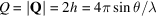, with sharp peaks where there are Bragg reflections and broad features in between. In general it is usual to work with a normalized version of this scattering intensity, S(Q). This is the intensity normalized by the incident flux per atom in the sample. S(Q) is called the total-scattering structure function. It is a dimensionless quantity and the normalization is such that the average value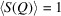. In short, S(Q) is nothing other than the powder diffraction pattern that has been corrected for experimental artifacts and suitably normalized (Egami & Billinge, 2013).

Measuring over a wide range of Q values yields better resolution in real space, as well as yielding more information, and is desirable. The coherent intensity (the features) in S(Q) dies out with increasing Q because of the Debye–Waller factor (which comes from thermal and quantum zero-point motion of the atoms), as well as any static displacive disorder in the material and, for X-ray measuerments, because of the X-ray form factor. In a neutron measurement, the atomic displacement effects are still present, but the neutron has no form factor and the scattering length is constant in Q. By a Q value of 30–50 Å−1 (depending on the temperature and the stiffness of the bonding in the sample) there are no more features in S(Q) and there is no need to measure data to higher Q. Still, this is a much higher maximum value of Q than is measured in conventional powder diffraction experiments using laboratory X-rays or reactor neutrons. The maximum value of Q attainable in back scattering from a Cu Kα tube is around 8 Å−1 and from an Mo Kα tube it is around 16 Å−1. Routine total-scattering measurements can be made using laboratory sources with Mo or Ag tubes; however, for the highest real-space resolution, and the smallest statistical uncertainties, synchrotron data are preferred. In the case of neutron scattering, spallation neutron sources are ideal for total-scattering experiments.

The total-scattering function S(Q) appears to be different from the function measured in a standard powder diffraction experiment because of the Q range studied, and also because of an important aspect of the normalization: the measured intensity is divided by the total scattering cross section of the sample. In the case of X-ray scattering, the sample scattering cross section is the square of the atomic form factor,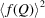, which becomes very small at high Q. Thus, during the normalization process the data at high Q are amplified (by being divided by a small number), which has the effect that even rather weak intensities at high Q, which are totally neglected in a conventional analysis of the data, become rather important in a total-scattering experiment. Because the signal at high Q is weak it is important to collect the data in that region with good statistics. This is illustrated in Fig. 1.1.22.Figure 1.1.22 | top | pdf |Comparison of raw data and the normalized reduced total-scattering structure function F(Q) = Q[S(Q) − 1]. The sample is a powder of 2 nm diameter CdSe nanoparticles and the data are X-ray data from beamline 6ID-D at the Advanced Photon Source at Argonne National Laboratory. The raw data are shown in the top panel. The high-Q data in the region Q > 9 Å−1 appear smooth and featureless. However, after normalizing and dividing by the square of the atomic form factor, important diffuse scattering is evident in this region of the diffraction pattern (bottom panel).

The Fourier transform of the total-scattering data is the reduced pair distribution function, G(r), which is related to S(Q) through a sine Fourier transform according to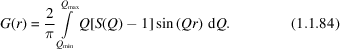Examples of G(r) functions from small nanoparticles of CdSe are shown in Fig. 1.1.23.Figure 1.1.23 | top | pdf |PDFs in the form of G(r) from bulk CdSe and from a series of CdSe nanoparticles. The blue curve at the bottom is the PDF obtained from the data shown in Figure 1.1.22. The blue symbols are from the data and the thin red lines on top are from models of the local structure in these nanoparticles. Offset below are difference curves between the model and the data. [Reprinted with permission from Masadeh et al. (2007). Copyright (2007) by the American Physical Society.]

G(r) has peaks at positions, r, that separate pairs of atoms in the solid with high probability. For example, there are no physically meaningful peaks below the nearest-neighbour peak at ∼2.5 Å, which is the Cd–Se separation in CdSe. However, in addition to the nearest-neighbour information, valuable structural information is contained in the pair correlations that extend to much higher values of r. In fact, with data to a high resolution in Q, PDFs can be measured out to hundreds of nanometres (i.e., thousands of ångströms) and the structural information that can be obtained from the data remains quantitatively reliable (Levashov et al., 2005).

The function G(r) is related to the atomic density. However, it is not the atomic density itself, but its autocorrelation. This is obtained by taking the atomic density of the molecule or cluster (which are the atoms at their respective positions) and convoluting it with a replica of the same thing. This object is then orientationally averaged to obtain the PDF. It is not a particularly intuitive object, but it is straightforward to calculate it from a given structural model. The inverse problem, calculating the structure from a PDF, is not possible directly, although in favourable cases, as with structure solution from powder diffraction, it is possible to obtain a unique structure solution from a PDF (Juhás et al., 2006).

We described above how to obtain G(r) from powder data. Here we briefly describe how to calculate a PDF from a structural model. To do this we have to introduce a related function to the PDF, the radial distribution function (RDF), R(r), which is related to G(r) by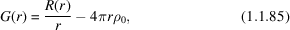where ρ0 is the atomic number density (Egami & Billinge, 2013).

The function R(r) is important because it is more closely related to the physical structure than G(r), since R(r) dr gives the number of atoms in an annulus of thickness dr at distance r from another atom. For example, the coordination number (or the number of neighbours) of an atom, NC, is given by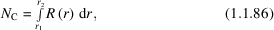where r1 and r2 define the start and end positions of the RDF peak corresponding to the coordination shell in question. This suggests a scheme for calculating PDFs from atomic models. Consider a model consisting of a large number of atoms situated at positions rν with respect to some origin. Expressed mathematically, this amounts to a series of delta functions, δ(rrν). The RDF is then given as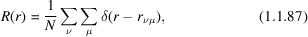where rνμ = |rνrμ| is the magnitude of the separation of the νth and μth ions, and the double sum runs twice over all atoms in the sample. In Chapter 5.7on PDF analysis we address explicitly samples with more than one type of atom, but for completeness we give here the expression for R(r) in this case: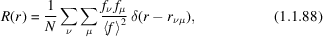where fν and fμ are the form factors, evaluated at Q = h = 0, for the νth and μth atoms, respectively, and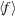is the sample-average form factor.

1.1.5.3.3. Inelastic coherent diffuse scattering

| top | pdf |

Scattering events must conserve energy and momentum. When a wave is scattered it changes direction and therefore changes its momentum. To satisfy conservation, this momentum, Q = 2πh = 2π(ss0), must be transferred to the material. When radiation is scattered by a crystal, the mass of the crystal is so large that this produces a negligible acceleration and the scattering is elastic. However, scattering from free atoms or fluids will produce a recoil, which results from a transfer of energy to the atom and the scattering is strictly inelastic. Even within a bulk crystal, there are lattice excitation modes (phonons) which may be created during a particular scattering event and the resulting scattering is inelastic. In an X-ray experiment, the energy resolution of the measurement usually is much too poor to separate this from the elastic scattering and it all appears mixed together (and is often simply referred to as elastic scattering'). As the excitation energies of internal modes of the system have energies of the order of meV (10−3 eV) and the X-ray energy is of the order of keV (103 eV), resolving the inelastic modes would require an energy resolution of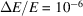, which is often unachievable. Nonetheless, such experiments are now carried out at synchrotron sources and provide important scientific insights, although the experiments are very slow and very specialized (Burkel, 1991).

These experiments are rarely carried out on powders. If the inelastic scattering is not resolved during the measurement, as is usually the case, it appears as a diffuse-scattering component in the signal from the powder or single crystal and it can be interpreted and modelled to extract information. In powder diffraction, when the scattering occurs from lattice vibrations, or phonons, the diffuse signal is called thermal diffuse scattering' or TDS (Warren, 1990). Over the last 50–60 years, a number of attempts have been made to extract information about phonon energies and phonon dispersions from TDS with varying amounts of success (Warren, 1990; Jeong et al., 1999; Graf et al., 2003; Goodwin et al., 2005). In the case of PDF analysis, the information in the TDS manifests itself in real space as correlated motion, and it is observed that the low-r peaks are sharper than the high-r peaks. This is because closely bonded atoms tend to move together: if an atom moves to the right it tends to push its neighbour also over to the right, so the motion is correlated. There is useful information in the TDS and the r dependence of the PDF peak broadening, but this is at best a very indirect way of measuring lattice-dynamical effects.

When the energy transfer is not resolved it is hard to separate the cases of scattering arising from phonons (which are dynamic atomic displacements) and scattering arising from static atomic displacements. To some extent these can be disentangled by studying the temperature dependence of the atomic displacement parameters (ADPs) obtained from modelling the data either in reciprocal space or real space (Billinge et al., 1991). This is often done by using a Debye model (Debye, 1912), where the temperature dependence of the mean-square ADP is given by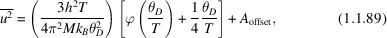where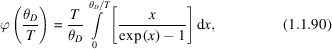is the Debye integral. Here,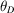is the Debye temperature, which is a measure of the stiffness of the bonding, h and kB are Planck's and Boltzmann's constants, respectively, and M is the mass of the oscillating atom. The constant Aoffset is a temperature-independent offset that is generally needed in the model to account for static distortions. The Debye model is rather crude but surprisingly useful and works well in many cases.

As in the case of phonons, if the scatterer couples to something else in the solid that has an excitation spectrum, this can be studied too. The case of neutrons scattered by magnetic moments is the best known example. Inelastic scattering gives direct information about the magnon dispersion curves. Information about magnetic excitations may also be obtained indirectly from the non-energy-resolved magnetic diffuse scattering signal. Magnetic PDF is now possible (Frandsen & Billinge, 2015) as described in Chapter 5.7, as well as reciprocal-space studies of magnetic diffuse scattering (Paddison & Goodwin, 2012).

1.1.5.3.4. Incoherent scattering

| top | pdf |

Incoherent scattering does not contain any structural information, and cannot be used to study structure in a diffraction experiment since the intensities of the scattered waves do not depend on the position of the scatterers. This does not mean that all incoherent scattering intensity is useless. The fluorescence intensity is incoherent, but may be used in EXAFS experiments to yield structural information. This is because coherent scattering of the photoexcited electron during an absorption event modulates the absorption cross section and therefore the incoherent fluorescence intensity, so a coherent scattering process leaves a measurable response in an incoherent intensity. Incoherent scattering can also be used to measure excitations, although all momentum-transfer information is lost so it is not possible to measure, for example, dispersions of excitations such as phonons and magnons. Even if the scattering process is incoherent, the energy exchanged between the probe and the sample can be measured by the change in wavelength of the scattered wave, and the amplitude of the scattering at each energy transfer is proportional to the density of states of the excitation being probed. In the case of neutrons, the very large incoherent cross section for scattering by hydrogen (∼100× the scattering cross section of most atoms) provides a strong signal for studying low-probability inelastic scattering events. Measuring inelastic scattering from powders can be a rapid way of determining the density of states of phonons, magnons and so on, which is very useful for determining the thermodynamic properties of materials, even though it is less precise than measurement of the full set of dispersion curves.

Another type of incoherent scattering that can be observed in X-ray experiments is Compton scattering (Compton, 1923; Cooper et al., 2004), which is an inelastic incoherent process where the scattering atom recoils during the scattering event. An example of Compton scattering measured in the spectrum from an energy-resolving detector is shown in Fig. 1.1.24.Figure 1.1.24 | top | pdf |Spectrum from an energy-resolving detector that shows the elastic and Compton signals as a function of scattering vector Q. [Reprinted with permission from Petkov et al. (2000). Copyright (2007) by the American Physical Society.]

The Compton scattering is strong in this experiment because the incident X-ray energy is high (80 keV) and the sample is a low-atomic-number alumina-silicate glass. Both the high X-ray energy and the low atomic numbers of the atoms in the sample increase the Compton cross section with respect to the coherent elastic scattering. As the magnitude of the scattering vector, Q, is increased the Compton scattering moves to lower energy and increases in intensity, but the elastic line stays fixed in energy and its intensity decreases because of form-factor and Debye–Waller effects. Momentum as well as energy is conserved in this process and the Compton scattering can be used to measure the momentum distribution of electrons in a material, although this kind of experiment is not widespread these days.

Elastic incoherent scattering provides no information about the sample, and simply degrades the signal-to-noise ratio of the measurement. As such, it is just inconvenient and cannot be easily removed. Monotonic Laue diffuse scattering originates from different chemical species with different scattering powers residing on different sites in the crystal, and when a destructive interference condition is satisfied the resulting intensity does not go to zero but is proportional to [fi(h) − fj(h)]2 (Warren, 1990). In pure elements in an X-ray experiment, the atoms on every site are the same and there is no Laue diffuse scattering. This is not true in neutron experiments where different nuclei have different scattering powers and most elements contain a range of isotopes in their natural form (the natural abundance'; Squires, 1996). This results in Laue diffuse scattering even in an element, although it is normally not referred to in these terms but is encompassed by a so-called incoherent neutron cross section' that is defined and tabulated (see Table 4.4.4.1in International Tables for Crystallography, Volume C) for each element. This is not the only source of incoherent scattering in neutron diffraction, since the scattering power also depends on the relative orientation of the neutron and nuclear spins. In general these spins are all orientationally disordered (and fluctuating) and the result is an additional scattering-event-dependent contribution to the incoherent scattering from the sample, again encompassed by the incoherent neutron cross section' of the element. Where necessary, it may be possible to make isotopically enriched samples for neutron experiments so that the proportion of isotopes with large incoherent scattering cross sections is minimized (or the isotopes are removed altogether), and the range of isotopes can also be reduced, which further reduces the incoherent component of the signal. However, the cost and difficulty of doing this means that it is rarely done.

References

Billinge, S. J. L., Davies, P. K., Egami, T. & Catlow, C. R. A. (1991). Deviations from planarity of copper-oxygen sheet in Ca0.85Sr0.15CuO2. Phys. Rev. B, 43, 10340–10352.Google Scholar
Burkel, E. (1991). Inelastic Scattering of X-rays With Very High Energy Resolution. Springer Tracts in Modern Physics. Amsterdam: Springer-Verlag.Google Scholar
Compton, A. H. (1923). A quantum theory of the scattering of X-rays by light elements. Phys. Rev. Lett. 21, 483.Google Scholar
Cooper, M. J., Mijnarends, P. E., Shiotani, N., Sakai, N. & Bansil, A. (2004). X-ray Compton Scattering. Oxford University Press.Google Scholar
Debye, P. (1912). Zur Theorie der spezifischen Wärmen. Ann. Phys. 344, 789–839.Google Scholar
Debye, P. (1915). Dispersion of Röntgen rays. Ann. Phys. 46, 809–823.Google Scholar
Egami, T. & Billinge, S. J. L. (2013). Underneath the Bragg Peaks: Structural Analysis of Complex Materials, 2nd ed. Amsterdam: Elsevier.Google Scholar
Frandsen, B. A. & Billinge, S. J. L. (2015). Magnetic structure determination from the magnetic pair distribution function (mPDF): ground state of MnO. Acta Cryst. A71, 325–334.Google Scholar
Goodwin, A. L., Tucker, M. G., Cope, E. R., Dove, M. T. & Keen, D. A. (2005). Model-independent extraction of dynamical information from powder diffraction data. Phys. Rev. B, 72, 214304.Google Scholar
Graf, M. J., Jeong, I. K., Starr, D. L. & Heffner, R. H. (2003). Limits on phonon information extracted from neutron pair-density functions. Phys. Rev. B, 68, 064305.Google Scholar
Jeong, I.-K., Proffen, T., Mohiuddin-Jacobs, F. & Billinge, S. J. L. (1999). Measuring correlated atomic motion using X-ray diffraction. J. Phys. Chem. A, 103, 921–924.Google Scholar
Juhás, P., Cherba, D. M., Duxbury, P. M., Punch, W. F. & Billinge, S. J. L. (2006). Ab initio determination of solid-state nanostructure. Nature, 440, 655–658.Google Scholar
Levashov, V. A., Billinge, S. J. L. & Thorpe, M. F. (2005). Density fluctuations and the pair distribution function. Phys. Rev. B, 72, 024111.Google Scholar
Paddison, J. A. M. & Goodwin, A. L. (2012). Empirical magnetic structure solution of frustrated spin systems. Phys. Rev. Lett. 108, 017204.Google Scholar
Squires, G. L. (1996). Introduction to the Theory of Thermal Neutron Scattering. New York: Dover Publications.Google Scholar
Warren, B. E. (1990). X-ray Diffraction. New York: Dover Publications.Google Scholar